# Ricci identity

(diff) ← Older revision | Latest revision (diff) | Newer revision → (diff)

An identity expressing one of the properties of the Riemann tensor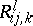(or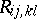):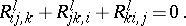For a covariant tensorthe identity is of the formi.e. cycling over the three first indices yields zero.

An identity which should be satisfied by the covariant derivatives of second order with respect to the metric tensor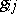of a Riemannian space, which differ only by the order of differentiation. If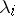is a tensor of valency 1 and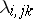is the covariant derivative of second order with respect to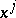andrelative to the tensor, then the Ricci identity takes the form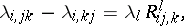whereis the Riemann curvature tensor determined by the metric tensorof the space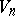(in other words, an alternating second absolute derivative of the tensor fieldin the metricis expressed in terms of the Riemann tensor and the components of).

For a covariant tensor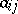of valency 2 the Ricci identity has the form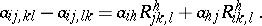In general, for a covariant tensor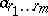of valencythe identity has the form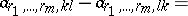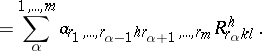Similar identities can be written for contravariant and mixed tensors in. The Ricci identity is used, e.g., in constructions of the geometry of subspaces inas an integrability condition for the principal variational equations from which Gauss' equations and the Peterson–Codazzi equations for subspaces ofare derived.

The identity was established by G. Ricci (see ).

How to Cite This Entry:
Ricci identity. Encyclopedia of Mathematics. URL: http://encyclopediaofmath.org/index.php?title=Ricci_identity&oldid=14933
This article was adapted from an original article by L.A. Sidorov (originator), which appeared in Encyclopedia of Mathematics - ISBN 1402006098. See original article